EECS2011 EECS 2011: Franck van Breugel's CodePages 136-140 (Section 4.1.1), pages 140-145 (Section 4.1.2), pages 149-152 (Section 4.2.1), pages 152-155 (Section 4.2.2) and pages 100-103 (Section 3.2)

Review pages 76-84 (Section 2.3 and 2.4)

Implementation of a stack and queue with an array in pseudocode: PostScript and PDF

Pages 163-165 (Section 4.3.2 and 4.3.3)

Review pages 159-163 (Section 4.3.1)

Implementation of a stack and queue with a singly linked list in pseudocode: PostScript and PDFPages 166-173 (Section 4.4), pages 184-186 (Section 5.1.1)

Implementation of a deque with a doubly linked list in pseudocode: PostScript and PDF

#### Question

Write a method that reverses a linked list.
```/**

@param node First node of the linked list to be reversed.
@return First node of the reversed list.
*/
public static Node reverse(Node node)
```Pages 187-193 (Section 5.1)

Review pages 111-118 (Section 3.5 and 3.6.1)

Implementation of a vector with an array in pseudcode: PostScript and PDF

#### Question

The question in PostScript and PDF.

Pages 108-109 (part of Section 3.4.3), pages 194-205 (Section 5.2)

Implementation of a list by means of a double linked list in pseudocode: PostScript and PDF

#### Question

Given a `List` whose elements are one of the following objects
```  public static final Object RED = new Object();
public static final Object WHITE = new Object();
public static final Object BLUE = new Object();
```
The list may contain each object more than once. Write a method
```  public static void sort(List list)
```
that sorts the elements: first all the red, then the white and finally the blue objects.

Pages 206-211 (Section 5.3), pages 211-214 (Section 5.4), pages 109-110 (part of Section 3.4.3), pages 214-218 (Section 5.5 and 5.6)

Implementation of a list with a circular array in pseudocode: PostScript and PDF

#### Loop invariants

Pre- and postconditions are predicates. Both tell us something about the values of the variables of our programs. For example, `n = 1' and `list is nonempty' are such predicates. We use
{ P } S { Q }
to specify that
if the variables have values such that the predicate P is satisfied at the start of the execution of statement S
and the execution of the statement S terminates
then at termination the variables have values such that the predicate Q is satisfied.
For example,
{ n = 0 } n = n + 1; { n = 1 }
and
{ true } list.insertLast("x"); { list is nonempty }

A loop invariant is also a predicate. It tells us something about the values of the variables while executing a loop. This predicate should be satisfied at the beginning of every iteration of the loop. Consider the loop
while B do S
with loop invariant LI. If
{ LIB } S { LI },
that is, statement S preserves the loop invariant LI within the loop, then we can conclude that
{ LI } while B do S { LIB },
that is, if the loop invariant LI is satisfied at the start of the execution of the loop and the loop terminates then the loop invariant LI (and of course alsoB) is satisfied at termination.

Consider the following Java snippet.

```int i = 1;
int x = 1;
while (i < N)
{
x *= i + 1;
i++;
}
```
For the above loop, the assertion x = i!i <= N is a loop invariant.

Algorithm bubbleSort(sequence):
Input: sequence of integers sequence
Postcondition: sequence is sorted and contains the same integers as the original sequence
lengthlength of sequence
i0
while i < length do
j0
while j < length - 1 do
if jth element of sequence > (j+1)th element of sequence then
swap jth and (j+1)th element of sequence

The outer loop has loop invariant
(A k : length - i <= k < length : (A l : k < l < length : kth element of sequence <= lth element of sequence))
and the inner loop has loop invariant
(A k : length - i <= k < length : (A l : k < l < length : kth element of sequence <= lth element of sequence))(A k : 0 <= k < j : kth element of sequence <= jth element of sequence)

#### Question

Consider the array based implementation of a list. In this implementation, each position contains an element and an index. For which operations would the worst case running time change if we would not store the index in a position?Pages 228-236 (Section 6.1), pages 236-246 (Section 6.2)

InspectableTree
Tree

#### Question

Consider a binary trees whose nodes contain integers. We will call such a binary tree t a binary search tree if for each internal node n of t
• all the integers stored in the left subtree of n are smaller than or equal to the integer stored in n, and
• all the integers stored in the right subtree of n are greater than or equal to the integer stored in n.
Write an algorithm that tests if a binary tree is a binary search tree.

Algorithm isBST(t):
Input: binary tree whose nodes contains integers
Output: Is t a binary search tree?Pages 246-257 (Section 6.3, excluding Section 6.3.5)

Proof of Proposition 6.10 and 6.11 in PostScript and PDF.

Algorithm isBST(t):
Input: binary tree whose nodes contains integers
Output: Is t a binary search tree?
if t consists of a single node then
resulttrue
else
resultisBST(left subtree of t)isBST(right subtree of t)smallerOrEqual(left subtree of t, integer of root of t)greaterOrEqual(right subtree of t, integer of root of t)
return result

Algorithm smallerOrEqual(t, i):
Input: binary tree whose nodes contains integers; an integer
Output: (A n : n is a node of t : element of n <= i)
resultelement of root of t <= i
if t consists of more than one node then
resultresultsmallerOrEqual(left subtree of t, i)smallerOrEqual(right subtree of t, i)
return result

Algorithm greaterOrEqual(t, i):
Input: binary tree whose nodes contains integers; an integer
Output: (A n : n is a node of t : element of n >= i)
resultelement of root of t >= i
if t consists of more than one node then
resultresultgreaterOrEqual(left subtree of t, i)greaterOrEqual(right subtree of t, i)
return result

#### Question

What is the running time of smallerOrEqual, greaterOrEqual and isBST?Pages 263-271 (Section 6.4.1 and 6.4.2)

Implementation of a binary tree by means of an array in PostScript and PDF

#### Question

Give the pseudocode for removeAboveExternal in the implementation of a binary tree with an array.Pages 271-273 (Section 6.4.3 and 6.4.4)

Implementation of a general tree by means of a binary tree in PostScript and PDF

#### Question

Give the algorithm for the operation children (as part of the implementation of a general tree by means of a binary tree).Section 8.1 (pages 334-338), Section 9.1 (pages 380-385)

Implementation of a dictionary by means of a binary search tree in PostScript and PDF.

#### Question

Consider the following Java snippet.
```  Dictionary d = new ...();
d.insertItem(new Integer(2), new Object());
if (d.findElement(new Integer(3)) == Dictionary.NO_SUCH_KEY))
{
}
else
{
System.out.println("Found!");
}
```
Explain why we cannot use `Dictionary.NO_SUCH_KEY.equals(d.findElement(new Integer(3)))` instead of `d.findElement(new Integer(3)) == Dictionary.NO_SUCH_KEY)`.Section 9.1 (pages 386-392)

Comparator
IntegerComparator

"More output"

Algorithm isBST(tree):
Input: a binary tree whose nodes contains keys
Output: (Is tree a binary search tree?, minimal key stored in tree, maximal key stored in tree)
if tree has one node then
result(true, key of root of tree, key of root of tree)
else
(isBSTleft, minleft, maxleft)isBST(left subtree of tree)
(isBSTright, minright, maxright)isBST(right subtree of tree)
isBSTisBSTleftisBSTrightmaxleft <= key of root of tree <= minright
minmin(key of root of tree, minleft, minright)
maxmin(key of root of tree, maxleft, maxright)
result(isBST, min, max)
return result

"More input"

Algorithm isBST(tree, min, max):
Input: a binary tree whose nodes contains keys; key; key
Output: Is tree a binary search tree?(A node : node is a node of tree : min <= key of node <= max)
if tree has one node then
resultmin <= key of root of tree <= max
else
resultmin <= key of root of tree <= maxisBST(left subtree of tree, min, min(key of root of tree, max))isBST(right subtree of tree, max(min, key of root of tree), max)
return result

Implementation of a binary tree by means of an array

Algorithm removeAboveExternal(node):
Precondition: node is external and different from the root
Input: a node of the binary tree
Postcondition: node and its parent have been removed from the tree and the sibling of node takes the place of its parent
Output: the element of the parent of node
indexindex of node
parentindex div 2
elementelement of tree[parent]
siblingindex + 1 - 2 * (index mod 2)
move(sibling, parent)
sizesize - 2
return element

Algorithm move(old, new):
Precondition: old is the index of a child of the node with index new
Input: two indices of nodes
Postcondition: the subtree rooted at node with index old replaces the subtree rooted at node with index new
tree[new]tree[old]
if tree[old] is an internal node then
move(2 * old, 2 * new)
move(2 * old + 1, 2 * new + 1)
else
tree[2 * new]empty
tree[2 * new + 1]empty

#### Question

Implement removeAllElements(k).#### Midterm

The midterm will be held in CSE A on Wednesday June 25 from 18:00 until 20:00. More information about the midterm can be found here.Section 9.2 (pages 393-396)

Proof of Proposition 9.2 in PostScript and PDF

Algorithm removeAll(key):
Input: key
Output: collection of elements of items in tree whose key is key
Postcondition: items whose key is key have been removed from tree
collectionempty collection
removeAll(key, root of tree, collection)
return collection

Algorithm removeAll(key, node, collection):
Input: key; node of tree; collection of elements
Postcondition: elements of items in subtree rooted at node whose key is key have been added to collection and those items have been removed from subtree rooted at node
if node is not a leaf then
if key of node > key then
removeAll(key, left child of node, collection)
else if key of node < key then
removeAll(key, right child of node, collection)
else
removeAll(key, right child of node, collection)
elementremove(key, node)

#### Question

Draw a smallest (in the number of nodes) AVL tree of height 5.Section 9.2 (pages 396-399)

#### Question

Consider an AVL tree. An item is inserted. Let n1 be the first node on the path from the inserted node to the root of the tree that has become unbalanced because of the insertion. Below you find a picture of the subtree rooted at the node n1.Assume that the item has been inserted in the subtree T2.
• What is the balance factor of node n1?
• What is the balance factor of node n2?
• What is the balance factor of node n3?Section 9.2 (pages 400-403) and Section 7.1-7.2 (pages 286-301).

PriorityQueue

Implementation of a priority queue by means of an (un)sorted sequence in PostScript and PDF.

#### Question

If you need an implementation of a priority queue, would you use the implementation by means of an unsorted sequence or the one by means of a sorted sequence?Section 7.2.2 (pages 296-297) and Section 7.3-7.3.2 (pages 301-310)

Item
UnsortedSequencePriorityQueue
InvalidKeyException

Proof of Proposition 7.5 in PostScript and PDF

Assume we have the following heap.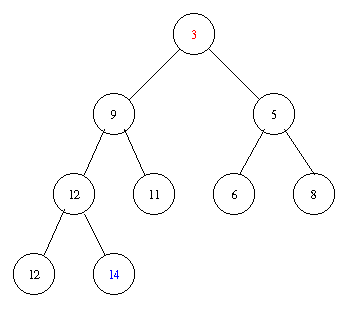Removing the minimal element takes the following steps.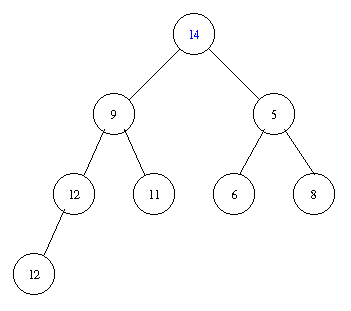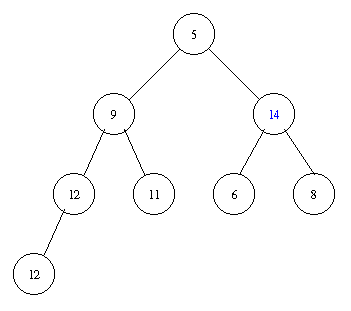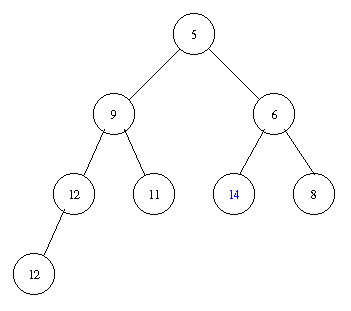Inserting an element with key 8 takes the following steps.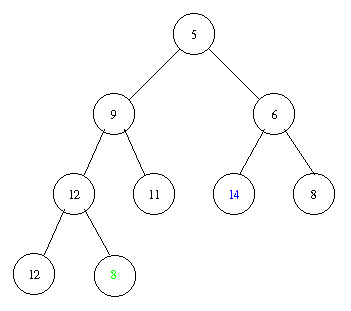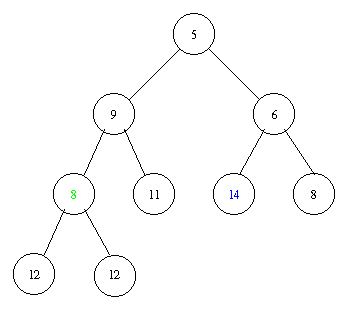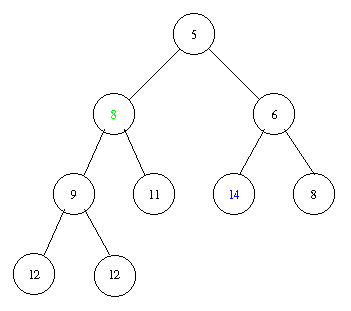Implementation of a priority queue with a heap in pseudocode: PostScript and PDF

#### Question

Consider the following Java method that returns the minimal element of a two-dimensional array of integers using priority queues.
```/**
Returns the minimum of the specified matrix.
The specified matrix is assumed to have the same number of rows as columns.

@param matrix a two-dimensional array of integers.
@return the minimum of the specified matrix.
*/
public static Integer minimum(Integer[][] matrix)
{
final int DIMENSION = matrix.length;
PriorityQueue minQueue = new ?PriorityQueue(new IntegerComparator());
for (int r = 0; r < DIMENSION; r++)
{
PriorityQueue rowQueue = new ?PriorityQueue(new IntegerComparator());
for (int c = 0; c < DIMENSION; c++)
{
rowQueue.insertItem(new Item(matrix[r][c], matrix[r][c]));
}
Object element = rowQueue.removeMin();
minQueue.insertItem(new Item(element, element));
}
return minQueue.removeMin();
}
```
The priority queues `minQueue` and `rowQueue` can be implemented either by a sorted sequence, an unsorted sequence or a heap. Which choice for these priority queues gives rise to the most efficient algorithm (the algorithm with the best worst case running time)? What is the worst case running time for those choices?Section 7.3.3-7.3.4 (pages 311-315), Section 8.3.1-8.3.2 (pages 342-343)

HeapPriorityQueue
PriorityQueueFullException
InPlaceHeapSorter

#### Question

Write a recursive algorithm to check if a binary tree (whose nodes contain items) is a heap.

Pages 343-350 (Section 8.3-8.3.5)

Implementation of a dictionary by means of a hash table: PostScript and PDF

#### Question

Assume we use the cyclic shift hash code and division map and that there are 101 buckets. In which bucket would the item ("key", "element") end up?

Pages 352-357 (Section 8.3-8.3.7) and pages 358-361 (Section 8.5)

Pseudocode for linear probing: PostScript and PDF

#### Question

Give an example of the quadratic probing strategy not finding an empty bucket even though there is one. You do not need more than five buckets.

Pages 360-361 (Section 8.5), pages 538-543 (Section 12.1)

A simple cycle is a path
• of which the first and last vertex are the same,
• all other vertices are different, and
• which has at least three different vertices.
A cycle is a path
• of which the first and last vertex are the same and
• which contains a simple cycle.
Proof of Proposition 12.11 in PostScript and PDF

#### Question

Consider the following simple undirected graph.• What are the endpoints of edge a?
• Are the vertices 1 and 3 adjacent?
• Is the edge c incident on 2?
• What is the degree of 2?
• Does the graph contain simple cycles? If so, give one.
• Does the graph contain cycles that are not simple? If so, give one.

Pages 543-546 (Section 12.1)

Proof of Proposition 12.11 in PostScript and PDF

Pages 547-553 (Section 12.2)

Implementation of a simple graph by means of an edge list: PostScript and PDF

Implementation of a simple graph by means of an adjacency list: PostScript and PDF

#### Question

Implement removeEdge using the edge list in O(1).

Pages 554-556 (Section 12.2)

Implementation of a simple undirected graph by means of an adjacency matrix: PostScript and PDF

In the implementation of a graph by means of an adjacency matrix, we restrict our attention to undirected graphs. There are simple mixed graphs whose representation by means of an adjacency matrix give rise to a matrix in which cells contain more than one edge (Can you think of an example?).

#### Question

Write an algorithm that tests if a simple undirected graph is connected.